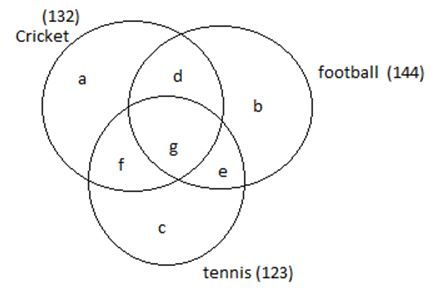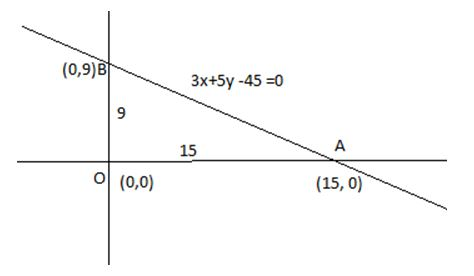# CAT 2019 – Slot 1 – Quantitative Ability – With rectangular axes of coordinates, the number of paths from (1,1) to (8,10) via (4,6)

Q. 1: With rectangular axes of coordinates, the number of paths from (1,1) to (8,10) via (4,6), where each step from any point (x, y) is either to (x, y+1) or to (x+1, y), is

dimension of rectangle with ends of diagonal being(1,1) and (4,6) = 3*5

thus number of paths from (1,1) to (4,6) = (5+3)!/5!3! =56

dimension of rectangle with ends of diagonal being(8,10) and (4,6) = 4*4

thus number of paths from (4,6) to (8,10) = (4+4)!/4!4! =70

so total number of paths from (1,1) to (8,10) via (4,6) = 56*70 =3920

Q 2: Amala, Bina, and Gouri invest money in the ratio 3 : 4 : 5 in fixed deposits having respective annual interest rates in the ratio 6 : 5 : 4. What is their total interest income (in Rs) after a year, if Bina’s interest income exceeds Amala’s by Rs 250?
1.7250
2.6350
3.7000
4.6000

Ratio of their interest at the end of a year = 3*6 : 4*5 : 5*4 = 18 : 20:20

As per question 20x -18x = 250

2x = 250

X = 125

So total interest = (18+20+20)*x = 58*125 = 7250

Q. 3: A club has 256 members of whom 144 can play football, 123 can play tennis, and 132 can play cricket. Moreover, 58 members can play both football and tennis, 25 can play both cricket and tennis, while 63 can play both football and cricket. If every member can play at least one game, then the number of members who can play only tennis is
1. 32
2. 38
3. 43
4. 45As per question a+b+c + (d+e+f) + g =256—————-1)

From figure , a+b+c + 2(d+e+f) + 3g = 132+144+123 = 399 ———-2)

Also g+e = 58 ———x)

f+g = 25—————y)

and d+g = 63————z)

adding all three , d+e+f + 3g = 58+25 + 63 = 146 ————-3)

from eq 2) and 3) , a+b+c + d+e+f = 399 – 146

a+b+c + d+e+f = 253————4)

from eq 1 and eq 4) g = 256 – 253 =3

so from eq x) , y) and z) d= 60, e = 55 and f = 22

Number of people playing tennis = 123

So g + f + e + c = 123

3+22 + 55 + c = 123

Or c = 43

the number of members who can play only tennis = 43

Q. 4: Let T be the triangle formed by the straight line 3x + 5y – 45 = 0 and the coordinate axes. Let the circumcircle of T have radius of length L, measured in the same unit as the coordinate axes. Then, the integer closest to L is

given eq of straight line is 3x + 5y – 45 = 0,
the line cuts x axis at
Put y = 0, 3x – 45 = 0 or x = 15 , so (15, 0)
Put x = 0, 5y – 45= 0 ,or y = 9
Thus the line cut y –axis at (0,9)Thus we can say OAB is the triangle T which is right angled triangle so AB will be dia of triangle.

Diameter AB = (9^2 + 15^2)^1/2 = (81+225)^1/2 = (306)^1/2 = 17.54 (approx)
So radius = 17.54/2 = 9 (approx)

Q. 5: Three men and eight machines can finish a job in half the time taken by three machines and eight men to finish the same job. If two machines can finish the job in 13 days, then how many men can finish the job in 13 days?

Let a man can finish the job alone in M days.

Given two machines can finish the job in 13 days so one machine can finish the job in 13*2 = 26 days

As given that Three men and eight machines can finish a job in half the time taken by three machines and eight men to finish the same job

So 3/M + 8/26 = 2(8/M + 3/26)

3/M + 4/13 = 16/M + 3/26

16/M – 3/M = 4/13 – 3/13

13/M = 1/13

M = 169

##### Checkout Other Questions of CAT 2019 Slot 1 Paper:

Verbal Ability :              |   Q.01- Q.05  |  Q.06- Q.10  |  Q.11- Q.15  |  Q.16- Q.20  |  Q.21- Q.24  |  Q.25- Q.29  |  Q.30 – Q.34  |

Logical Reasoning :    |   Q.29 – Q.32  |

Quantitative Aptitude: |

### Free Material Area